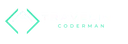Back to main page# Declaring over updating RxJS observables

With RxJS Observables, there are two ways to compute new observables from existing ones.

## Updating 🔗

In the first way, you update the computed observable each time one of the sources change.

Let’s take the example of addition. Two numbers exist that can each change independently. A third number should be computed as the sum of both numbers.

In an updating approach, we emit a new sum value each time one of the sources change. With two numbers, that is still realistic. If an observable is computed though from a variety of different sources, then it can be easy to forget emitting a new value for the computed observable.

``number1\$ = new BehaviorSubject<number>(0);number2\$ = new BehaviorSubject<number>(0);sum\$ = new BehaviorSubject<number>(0);incrementFirstNumber() {  number1\$.next(number1\$.value + 1);  sum\$.next(number1\$.value + number2\$.value);}incrementSecondNumber() {  number2\$.next(number2\$.value + 1);  sum\$.next(number1\$.value + number2\$.value);}``

## Declaring 🔗

A different, preferred, approach requires you to declare what the sum is. Then RxJS takes care of updating it each time one of the sources change.

While the declaration looks a bit more complex, it doesn’t require updating the sum after one of the numbers has changed.

``number1\$ = new BehaviorSubject<number>(0);number2\$ = new BehaviorSubject<number>(0);sum\$: Observable<number>;ngOnInit() {	sum\$ = combineLatest([number1\$, number2\$])		.pipe(			map(([number1, number2]) => number1 + number2),			shareReplay(1)		);}incrementFirstNumber() {  number1\$.next(number1\$.value + 1);}incrementSecondNumber() {  number2\$.next(number2\$.value + 1);}``

Note: The expression `shareReplay(1)` is helpful to ensure that the computed observable has a value.

## Angular-specific outlook: Signals 🔗

With the upcoming Angular signals, declaring computed values becomes simpler.

Note the simpler syntax. Also, how the sum is guaranteed to always have a value, which was not the case with RxJS observables.

``number1 = signal(0);number2 = signal(0);sum = computed(() => number1() + number2());incrementFirstNumber() {  number1.update((value) => value + 1);}incrementSecondNumber() {  number2.update((value) => value + 1);}``Fabian Böller

Product-minded Fullstack Engineer